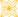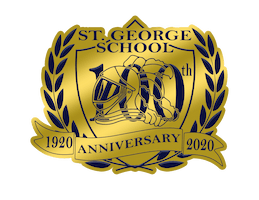# St. George School# Curriculum Guidelines

Geometry

The geometry skills and concepts developed in this discipline are useful to all
students. Aside from learning these skills and concepts, students will develop
their ability to construct formal. Logical arguments and proofs in geometric
settings and problems.

1.0 Students demonstrate understanding by identifying and giving examples of
undefined terms, axioms, theorems, and inductive and deductive reasoning.

2.0 Students write geometric proofs, including proofs by contradiction. If a line L
is tangent to a circle at a point P, prove that the radius passing through P is
perpendicular to L.

3.0 Students construct and judge the validity of a logical argument and give
counterexamples to disprove a statement.

4.0 Students prove basic theorems involving congruence and similarity.

5.0 Students prove that triangles are congruent or similar, and they are able to
use the concept of corresponding parts of congruent triangles.

6.0 Students know and are able to use the triangle inequality theorem.

7.0 Students prove and use theorems involving the properties of parallel lines
cut by a transversal, the properties of quadrilaterals, and the properties of
circles.

8.0 Students know, derive and solve problems involving the perimeter,
circumference, area, volume, lateral area, and surface area of common
geometric figures.

9.0 Students compute the volumes and surface areas of prisms, pyramids,
cylinders, cones, and spheres; and student commit to memory the formulas
for prisms, pyramids, and cylinders.

10.0 Students compute areas of polygons, including rectangles, scalene triangles,
equilateral triangles, rhombi, parallelograms, and trapezoids.

11.0 Students determine how changes in dimensions affect the perimeter, area
and volume of common geometric figures and solids.

12.0 Students find and use measures of sides and of interior and exterior angles
of triangles and polygons to classify figures and solve problems.

13.0 Students prove relationships between angles in polygons by using
properties of complementary, supplementary, vertical, and exterior angles.

14.0 Students prove the Pythagorean Theorem.

15.0 Students use the Pythagorean Theorem to determine distance and find
missing lengths of sides of right triangles.

16.0 Students perform basic constructions with a straightedge and compass, such
as angle bisectors, perpendicular bisectors, and the line parallel to a given
line through a point off the line.

17.0 Students prove theorems by using coordinate geometry, including the
midpoint of a line segment, the distance formula, and various forms of
equations of lines and circles.

18.0 Students know the definitions of the basic trigonometric functions defined by
the angles of a right triangle. They also know and are able to use
elementary relationships between them for example, tan(x) = sin(x)/cos(x),
(sin(x))² + (cos(x))² = 1.

19.0 Students use trigonometric functions to solve for an unknown length of a side
of a right triangle, given an angle and a length of side.

20.0 Students know and are able to use angle and side relationships in problems
with special right triangles, such as 30°, 60°, and 90° triangles and 45°, and
90° triangles.

21.0 Students prove and solve problems regarding relationships among chords,
secants, tangents, inscribed angles, and inscribed and circumscribed
polygons of circles.

22.0 Students know the effect of rigid motions on figures in the coordinate plane
and space, including rotations, translations, and reflections.

Algebra II

This discipline complements and expands the mathematical content and
concepts of Algebra I and geometry. Student who mater Algebra II will gain
experience with algebraic solutions of problems in various content areas,
including the solution systems of quadratic equations, logarithmic and
exponential functions, the binomial theorem, and the complex number system.

1.0 Students solve equations and inequalities involving absolute value.

2.0 Students solve systems of linear equations and inequalities (in two of three
variables) by substitution, with graphs, or with matrices.

3.0 Students are adept at operations on polynomials, including long division.

4.0 Students factor polynomials representing the difference of squares, perfect
square trinomials, and the sum and difference of two cubes.

5.0 Students demonstrate knowledge of how real and complex numbers are
related both arithmetically and graphically. In particular, they can plot
complex numbers as points in the plane.

6.0 Students add, subtract, multiply and divide complex numbers.

7.0 Students add, subtract, multiply, divide, reduce, and evaluate rational
expressions with monomial and polynomial denominators and simplify
complicated rational expressions, including those with negative exponents
in the denominator.

8.0 Students solve and graph quadratic equations by factoring, completing the
square, or using the quadratic formula. Students apply these techniques in
solving word problems. They also solve quadratic equations in the complex
number system.

9.0 Students demonstrate and explain the effect that changing a coefficient has
on the graph of quadratic functions: that is, students can determine how the
graph of a parabola changes as a, b, and c vary in the equation
y = a(x-b)² + c.

10.0 Students graph quadratic functions and determine the maxima, minima, and
zeros of the function.

11.0  Students prove simple laws of logarithms.

11.1 Students understand the inverse relationship between exponents
and logarithms and use this relationship to solve problems involving
logarithms and exponents.

11.2 Students judge the validity of an argument according to whether the
properties of real numbers, exponents, and logarithms have been
applied correctly at each step.

12.0 Students know the laws of fractional exponents, understand exponential
functions, and use these functions in problems involving exponential growth
and decay.

13.0 Students use the definition of logarithms to translate between logarithms in
any base.

14.0 Students understand and use the properties of logarithms to simplify
logarithmic numeric expressions and to identify their approximate values.

15.0 Students determine whether a specific algebraic statement involving rational
expressions, radical expressions, or logarithmic or exponential functions is
sometimes true, always, true, or never true.

16.0 Students demonstrate and explain how the geometry of the graph of a conic
section (e.g. asymptotes, foci, eccentricity) depends on the coefficients of

17.0 Given a quadratic equation of the form ax² +by² + cx + dy + e = 0, students
can use the method for completing the square to put the equation into
standard form and can recognize whether the graph of the equation is a
circle, ellipse, parabola, or hyperbola. Students can graph the equation.

18.0 Students use fundamental counting principles to compute combinations and
permutations.

19.0 Students use combinations and permutations to compute probabilities.

20.0 Students know the binomial theorem and use it to expand binomial
expressions that are raised to positive integer powers.

21.0 Students apply the method of mathematical induction to prove general

22.0 Students find the general term and the sums of arithmetic series and for both
finite and infinite geometric series.

23.0 Students derive the summation formulas for arithmetic series and for both
finite and infinite geometric series.

24.0 Students solve problems involving functional concepts, such as composition,
defining the inverse function and performing arithmetic operations on
functions.

25.0 Students use properties from number systems to justify steps in combining
and simplifying functions.

Catholic Students Today - Catholic Leaders Tomorrow - Estudiantes Católicos Hoy- Lideres Católicos Mañana